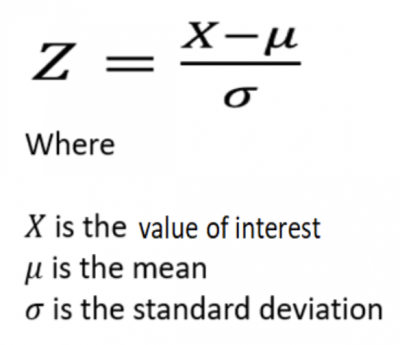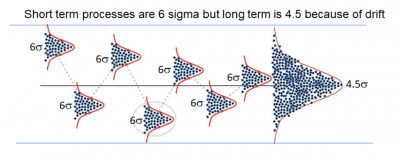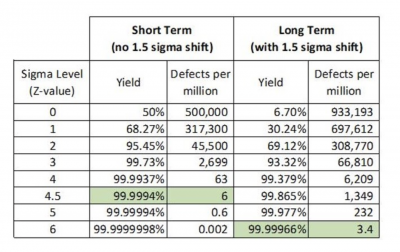Z shift by definition is the difference between long-term Z and short-term Z. In this article, we will define Z in all its forms, including the statistical foundation, calculations, benefits, and best practices of using the Z shift to describe your process capability.

## Overview: What is Z shift?

Before explaining Z shift, you should understand the following concepts: Z distribution, Z score, Z table, Z.bench, Z short-term, and Z long-term.

1. Z distribution: The Z-distribution, also known as the standard normal distribution, is a special normal distribution where the mean is 0 and the standard deviation is 1. Converting and standardizing a normal distribution allows you to calculate the probability of certain values occurring.
2. Z score: The Z score is the number of standard deviations a value of interest is away from the mean of a normal distribution. The formula for calculating Z score is.Z score
3. Z table: This is a table of Z scores that enables you to calculate the probabilities of a value of interest occurring. This is also known as the standard normal table.
4. Z.bench: Z.bench is a calculation of your process capability. It can be used as a substitute or complement to Ppk or Cpk. Z.bench is the z-value you get from a standard normal distribution if you place the probability of defects entirely on the right tail.
5. Long-term Z.bench: This is calculated using the overall or between standard deviation of the process. Because of drift over time, it is a better representation of reality. It’s the actual quality the customer receives in the long term.
6. Short-term Z.bench: This is calculated using the within-standard deviation of the process. It represents the quality your customer would get if you could keep the short-term variation constant.

Now that you understand the different versions of Z, you are ready to learn about the Z shift.

Z shift is the difference between long- and short-term Z.bench. The larger the shift, the more your process is drifting and more between variation you are experiencing. You might be doing well in the short term, but your customers are feeling the shifting variation in the long term.

While it is best to calculate the actual Z shift for your process, some Lean Six Sigma practitioners suggest using a standard shift of 1.5 between your long- and short-term Z.bench. This would be calculated as:

Z.bench (short) = Z.bench (long) + 1.5

The reasoning behind the 1.5 shift developed at Motorola was the realization that processes will deteriorate, drift, or shift in performance over time. This can be caused by changes in raw materials, equipment, people, technology, and other manufacturing changes that occur over time.

After years of data collection and empirical analysis, Motorola consultants Dr. Mikel Harry and Richard Schroeder came to the conclusion that the mean of the standard distribution will typically shift about 1.5 sigma. While a process may be performing at a Six Sigma level in the short term, the long-term process may only be operating at 4.5 sigma level. This became the definition of a Six Sigma process.

You can see the concept of the 1.5 shift with this graph:You can see the shift statistically in this graph:1.5 Sigma Shift

Notice that the short-term yield at 4.5 sigma (99.9994%) is essentially the same as the long-term yield for 6 sigma if you apply the 1.5 sigma shift (99.99966%). Also notice the defects per million opportunities is 3.4, which is used in the definition of Six Sigma.

Summing up the concept of the 1.5 sigma shift, Dr. Mikel Harry said this in the Forward of his book entitled Resolving the Mysteries of Six Sigma:

“…it should be fairly evident that the 1.5 sigma shift factor can often be treated as a “statistical correction,” but only under certain engineering conditions that would generally be considered “typical.” By all means, the shift factor (1.5 sigma) does not constitute a “literal” shift in the mean of a performance distribution — as many quality practitioners and process engineers falsely believe or try to postulate through uniformed speculation and conjecture … In summary, the 1.5 sigma shift factor should only be viewed as a mathematical construct of a theoretical nature. When treated as a “statistical correction,” its origin can be mathematically derived as an equivalent quantity representing or otherwise reflecting the “worst-case-error” inherent to an estimate of short-term process capability.”

## 3 benefits of Z shift

Some of the benefits of knowing the Z shift of your process include the following.

### 1. Measure of process capability

The computations for long- and short-term Z.bench and the resulting Z shift can be a useful measure of process capability equivalent to Ppk and Cpk.

### 2. Accounts for the reality of a shifting process

Processes have a tendency to shift and drift over time. Z shift provides an indication of your process stability.

### 3. Can be converted to defect level

Z.bench calculations can be converted to such calculations as defects per million opportunities (DPMO).

## Why is Z shift important to understand?

While Z shift is just the difference between Z.bench long and short term variation, it is important to understand the concepts behind the Z calculations.

### The 1.5 sigma shift is not an absolute

This correction factor may be used, but the true shift of your process is what’s important to know.

### All Z calculations are based on a normal distribution

Z values are a function of the standard normal distribution. For a non-normal distribution, the values will need to be transformed into a normal distribution so the standard normal distribution can be used.

### The larger the shift, the more opportunity for improvement

Z shift describes the shift, drift, and inconsistency of your process over time. Knowing the degree of shift you have will give you an indication of how much improvement you need to achieve.

## An industry example of Z shift

The head of the quality department calculated her company’s process capability on a monthly basis. Her calculations showed good Cpk and Z.bench values. Yet, when she surveyed her customers at the end of the year, they were not as satisfied as she thought they should be.

She decided to also start calculating Ppk and the Z.bench shift. Both showed the process was shifting and drifting over the course of the year. While her short-term variation was very good, the longer-term variation was less due to the process changes. The actual Z shift was 1.3, which was not acceptable to her and her customers.

An improvement team was formed to address the variation. The sources of the variation included variation in raw materials and equipment settings. Changes were made, and the actual shift was reduced to 0.56.

## 3 best practices when thinking about Z shift

### 1. Conduct a Measurement System Analysis (MSA)

For your Z calculations to be useful, you should conduct an MSA to be confident that your data accurately reflects your true process.

### 2. Be sure your process is stable

Control chart your data to be sure your process is only exhibiting common cause variation.

### 3. If possible, compute your own Z shift

The 1.5 sigma shift is a hypothetical correction factor. Calculate your own process Z shift if possible.

### 1. What is the calculation for Z shift?

It is Z.bench short term minus Z.bench long term. If you are using the 1.5 sigma shift, Z shift short term equals Z shift long term plus 1.5.

### 2. Who developed the concept of the 1.5 sigma shift?

Bill Smith, an engineer with Motorola, originated the idea of the 1.5 sigma shift as well as the concept of Six Sigma as design margins for the company’s electronic components.

### 3. What is a Z value

Z value is the number of standard deviations a value of interest is away from the mean of a distribution typically found in a standard normal table.

## Recapping the Z shift

Z shift is the mathematical difference between your short-term and long-term Z.bench. The larger the Z shift, the more opportunity to improve the process by eliminating shifts and drifts in your process mean.

Some organizations define Z.bench short term as long-term Z.benchmark plus a 1.5 sigma shift. Using the 1.5 correction factor results in a process long-term Z.benchmark of 4.5, although it is defined as a Six Sigma process. It is best to directly measure your actual short-term and long-term Z.benchmark capability.